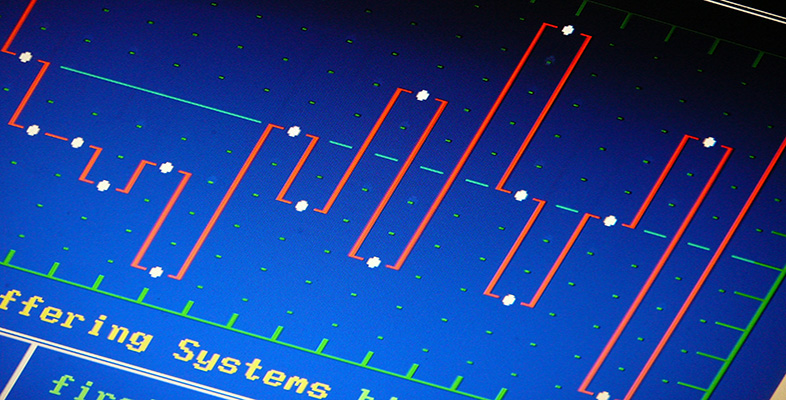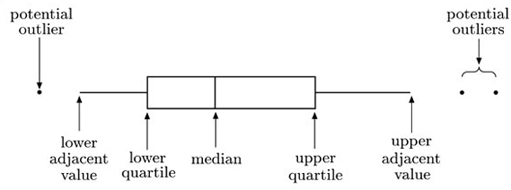Science, Maths & Technology

### Become an OU studentInterpreting data: Boxplots and tables

Start this free course now. Just create an account and sign in. Enrol and complete the course for a free statement of participation or digital badge if available.

# 1.5 Summary

In this section you have been introduced to the boxplot. This is a graphic that represents the key features of a set of data. A typical boxplot is shown in Figure 1.8.Figure 1.8 A typical boxplot

The ends of the box mark the quartiles, and the vertical line through the box is located at the median. The whiskers of a boxplot extend to values known as adjacent values. These are the values in the data that are furthest away from the median on either side of the box, but are still within a distance of 1.5 times the interquartile range from the nearest end of the box (that is, the nearer quartile). In many cases the whiskers actually extend right out to the most extreme values in the data set. However, in other cases they do not. Any values in the data set that are more extreme than the adjacent values are plotted as separate points on the boxplot. This identifies them as potential outliers that may need further investigation.

A boxplot depicts only some basic aspects of the distribution of the values in data set. But often these basic aspects are the ones of most interest. It is straightforward to draw boxplots of more than one data set on the same scale, and then to use them to compare important aspects of the distribution of the data sets. A systematic approach to carrying out such comparisons has been described.